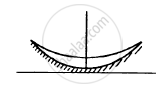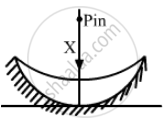Department of Pre-University Education, KarnatakaPUC Karnataka Science Class 12
Advertisement Remove all ads

# The Convex Surface of a Thin Concavo-convex Lens of Glass of Refractive Index 1.5 Has a Radius of Curvature 20 Cm. - Physics

Sum

The convex surface of a thin concavo-convex lens of glass of refractive index 1.5 has a radius of curvature 20 cm. The concave surface has a radius of curvature 60 cm. The convex side is silvered and placed on a horizontal surface as shown in figure. (a) Where should a pin be placed on the axis so that its image is formed at the same place? (b) If the concave part is filled with water (μ = 4/3), find the distance through which the pin should be moved so that the image of the pin again coincides with the pin.Advertisement Remove all ads

#### Solution

(a) Let F be the the focal length of the given concavo-convex lens. Then,
$\frac{1}{F} = \frac{1}{f_1} + \frac{1}{f_m} + \frac{1}{f_1}$
$= \frac{2}{f_1} + \frac{1}{f_m}$
$\left[ \because \frac{1}{f_m} = (\mu - 1)\left( \frac{1}{R_1} - \frac{1}{R_2} \right) \right]$$\frac{1}{F} = \frac{2}{30} + \frac{1}{15} = \frac{2}{15}$
Hence, R = 15 cm
Therefore, the pin should be placed at a distance of 15 cm from the lens.

(b) If the concave part is filled with water,
For focal length F'
$\frac{1}{F'} = \frac{2}{f_w} + \frac{2}{f_1} + \frac{1}{f_m}$
$= \frac{2}{90} + \frac{2}{30} + \frac{1}{15}$
$\left[ \because \frac{1}{f_w} = \left( \frac{4}{3} - 1 \right)\left( + \frac{1}{30} \right) \right]$
$\therefore F' = \frac{45}{7} cm$
Thus, pin should be placed at a distance of  $\frac{90}{7}$ cm from the lens.

Concept: Concave Mirror
Is there an error in this question or solution?
Advertisement Remove all ads

#### APPEARS IN

HC Verma Class 11, 12 Concepts of Physics 1
Chapter 18 Geometrical Optics
Q 47 | Page 415
Advertisement Remove all ads

#### Video TutorialsVIEW ALL 

Advertisement Remove all ads
Share
Notifications

View all notifications

Forgot password?